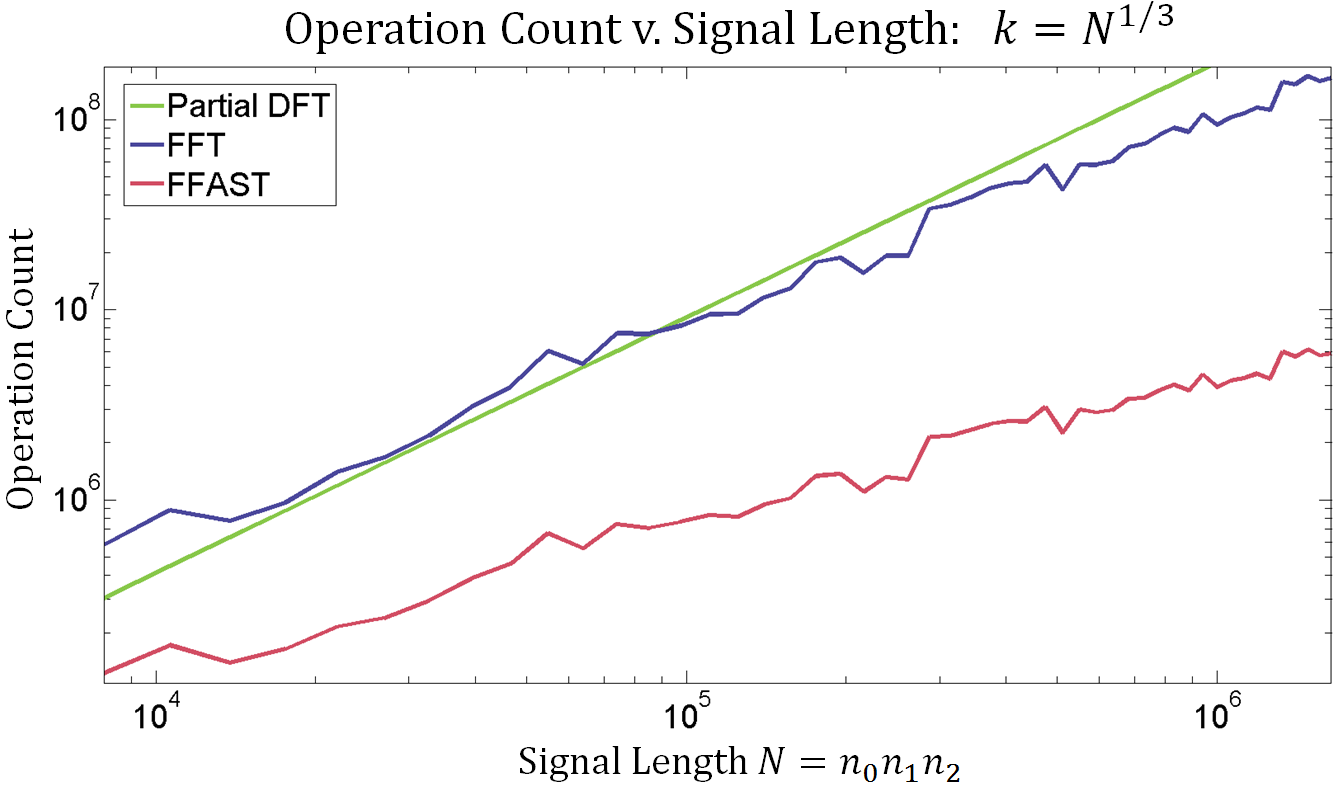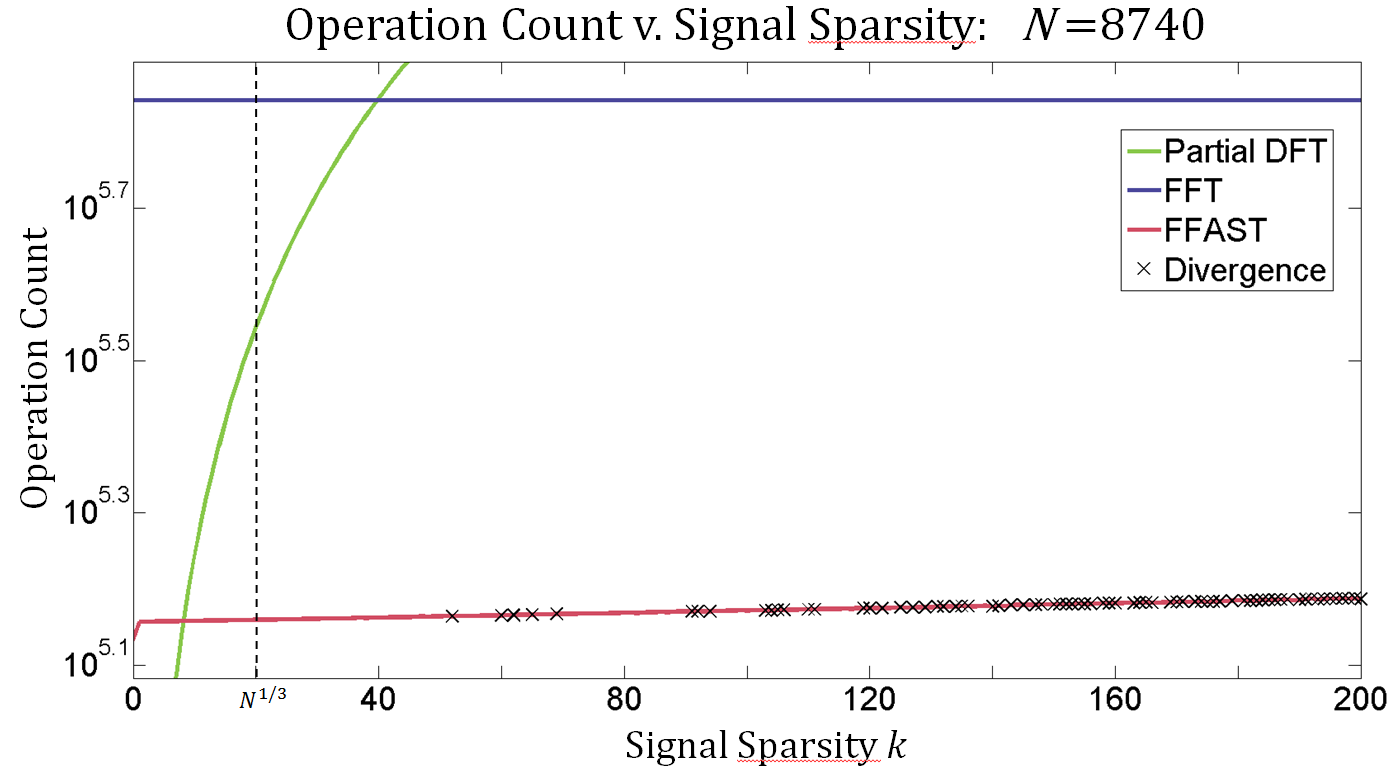# 0.4 Computational experiment of ffast

 Page 1 / 1

## Experiment design

We wanted to determine the most computationally efficient demodulation method for the digital multitone scheme. In our experiment, we compared the operation counts of an optimized FFT meta algorithm, a partial DFT computation (the DFT computed only for the nonzero coefficients), and FFAST in demodulating various message signals. We chose these demodulation methods because they are currently the most efficient methods for DMT demodulation. The experiments were run using MATLAB 2014a. We chose to run our experiment in MATLAB for its rapid prototyping environment.

While we were interested in comparing the computational efficiency of these algorithms, we chose to record operation counts rather than run times. Run times are unreliable metrics on machines with multitasking operating systems, especially when using highly optimized programs like MATLAB. We chose to count complex additions and multiplications as one operation each. We did not count conditional statements as operations because in most general processors, they only require a single cycle. We counted complex exponentials and trigonometric functions as one operation because they may be implemented using lookup tables.

## Meta-fft algorithm implementation

The two main goals for our implementation of the optimized FFT meta algorithm were to i) create an algorithm that performs without the need to zero-pad the signal and ii) allows us to count operations. Well-behaved signal lengths in our implementation of the algorithm have the form of $N={n}_{0}{n}_{1}{n}_{2}$ , where ${n}_{i}$ are coprimes. To exploit this structure, the optimized FFT meta algorithm, implemented in meta_fft.m , uses a self-sorting mixed-radix complex FFT [link] . For sub-transforms of length $2\le N\le 6$ , short-length Winograd transforms are applied to conserve operation count. All other transforms are computed using Rader's FFT algorithm [link] .

## Ffast implementation

The FFAST algorithm implementation requires the signal itself, the length of the signal, and a vector of the downsampling coefficients.

The FFAST algorithm was implemented in two files. The first file, ffast_front_end.m , downsamples the signal by each of the coprimes and feeds shifted and unshifted versions of the downsampling to the meta_fft.m file, to get operation counts and the relevant FFTs. Once the relevant DFT pairs are generated, ffast_front_end.m calls the back end of the algorithm.

The second file, peeling_decoder.m , implements the peeling module of FFAST to backsolve the bipartite graph. The program will return a flag if the algorithm encounters no singletons at a stage where it has not been fully solved.

## Numerical results

In our first experiment, we varied the signal length N and observed the operation counts required for the optimized FFT meta algorithm, the partial DFT, and FFAST. We constructed each signal in the Fourier domain by randomly selecting $k$ values from the set of integers $\left\{1,\cdots ,N\right\}$ and setting the corresponding DFT coefficients to $\frac{k}{2}$ . For each signal, we put $k={N}^{1/3}$ , which is the greatest allowed sparsity in our scheme. The choice of $k$ and values of the $k$ nonzero DFT coefficients are consistent with the DMT scheme. We used MATLAB's library function ifft() to compute the corresponding signal and counted the number of operations it took each algorithm to compute the DFT. We observed that the operation count required for FFAST was usually an order of magnitude less that the operation counts required for both the meta FFT algorithm and the partial DFT. See Fig  [link] for the results of the first experiment.Experiment 1

In our second experiment, we varied the signal sparsity $k$ and observed the operation count required for the optimized FFT meta algorithm, the partial DFT, and FFAST. For this experiment, signal length $N=8740$ and the signals were constructed in the Fourier domain, as before. We observed an $80%$ computational decrease from the optimized FFT to FFAST for all $k<{N}^{\frac{1}{3}}$ . However, for $k>{N}^{\frac{1}{3}}$ , we observed signals for which FFAST did not converge. See Fig  [link] for the results of the second experiment.Experiment 2

Note that the operation count for the partial DFT is less than the operation count for FFAST for $k<8$ ; this is somewhat misleading because the way that the partial DFT is computed is slightly optimistic. The partial DFT computes only the nonzero coefficients, which are known a priori in this experiment. In the general framework of DMT, one would need to compute all possibly nonzero DFT coefficients, resulting in an operation count higher than that of FFAST.

#### Questions & Answers

Is there any normative that regulates the use of silver nanoparticles?
Damian Reply
what king of growth are you checking .?
Renato
What fields keep nano created devices from performing or assimulating ? Magnetic fields ? Are do they assimilate ?
Stoney Reply
why we need to study biomolecules, molecular biology in nanotechnology?
Adin Reply
?
Kyle
yes I'm doing my masters in nanotechnology, we are being studying all these domains as well..
Adin
why?
Adin
what school?
Kyle
biomolecules are e building blocks of every organics and inorganic materials.
Joe
anyone know any internet site where one can find nanotechnology papers?
Damian Reply
research.net
kanaga
sciencedirect big data base
Ernesto
Introduction about quantum dots in nanotechnology
Praveena Reply
what does nano mean?
Anassong Reply
nano basically means 10^(-9). nanometer is a unit to measure length.
Bharti
do you think it's worthwhile in the long term to study the effects and possibilities of nanotechnology on viral treatment?
Damian Reply
absolutely yes
Daniel
how to know photocatalytic properties of tio2 nanoparticles...what to do now
Akash Reply
it is a goid question and i want to know the answer as well
Maciej
characteristics of micro business
Abigail
for teaching engĺish at school how nano technology help us
Anassong
Do somebody tell me a best nano engineering book for beginners?
s. Reply
there is no specific books for beginners but there is book called principle of nanotechnology
NANO
what is fullerene does it is used to make bukky balls
Devang Reply
are you nano engineer ?
s.
fullerene is a bucky ball aka Carbon 60 molecule. It was name by the architect Fuller. He design the geodesic dome. it resembles a soccer ball.
Tarell
what is the actual application of fullerenes nowadays?
Damian
That is a great question Damian. best way to answer that question is to Google it. there are hundreds of applications for buck minister fullerenes, from medical to aerospace. you can also find plenty of research papers that will give you great detail on the potential applications of fullerenes.
Tarell
what is the Synthesis, properties,and applications of carbon nano chemistry
Abhijith Reply
Mostly, they use nano carbon for electronics and for materials to be strengthened.
Virgil
is Bucky paper clear?
CYNTHIA
carbon nanotubes has various application in fuel cells membrane, current research on cancer drug,and in electronics MEMS and NEMS etc
NANO
so some one know about replacing silicon atom with phosphorous in semiconductors device?
s. Reply
Yeah, it is a pain to say the least. You basically have to heat the substarte up to around 1000 degrees celcius then pass phosphene gas over top of it, which is explosive and toxic by the way, under very low pressure.
Harper
Do you know which machine is used to that process?
s.
how to fabricate graphene ink ?
SUYASH Reply
for screen printed electrodes ?
SUYASH
What is lattice structure?
s. Reply
of graphene you mean?
Ebrahim
or in general
Ebrahim
in general
s.
Graphene has a hexagonal structure
tahir
On having this app for quite a bit time, Haven't realised there's a chat room in it.
Cied
what is biological synthesis of nanoparticles
Sanket Reply
how did you get the value of 2000N.What calculations are needed to arrive at it
Smarajit Reply
Privacy Information Security Software Version 1.1a
Good
Got questions? Join the online conversation and get instant answers!
Jobilize.com Reply

### Read also:

#### Get the best Algebra and trigonometry course in your pocket!

Source:  OpenStax, Using ffast to decrease computation time in digital multitone communication. OpenStax CNX. Dec 17, 2014 Download for free at http://legacy.cnx.org/content/col11731/1.1
Google Play and the Google Play logo are trademarks of Google Inc.

Notification Switch

Would you like to follow the 'Using ffast to decrease computation time in digital multitone communication' conversation and receive update notifications?By Naveen TomarBy